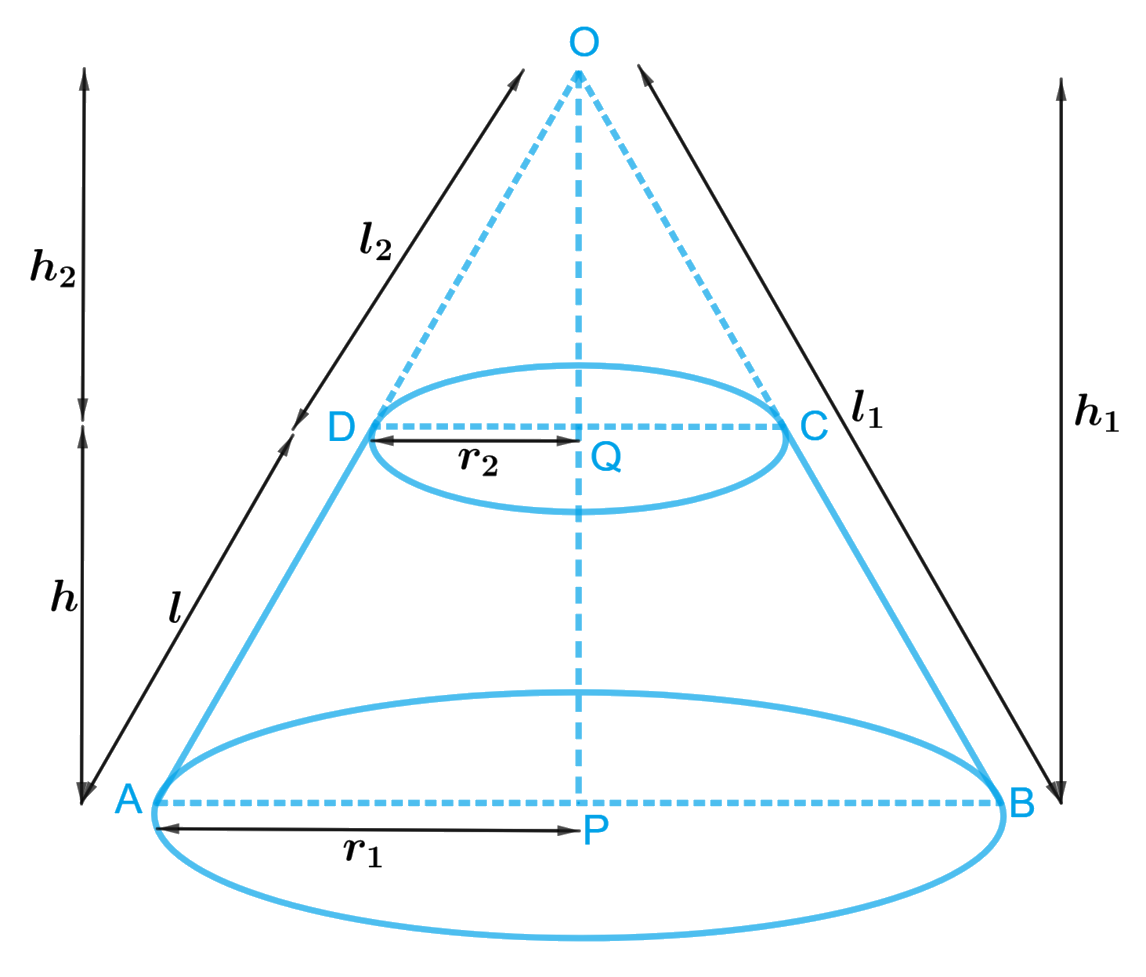# Ex.13.5 Q6 Surface Areas and Volumes Solution - NCERT Maths Class 10

Go back to  'Ex.13.5'

## Question

Derive the formula for the curved surface area and total surface area of the frustum of a cone, given to you in Section $$13.5$$, using the symbols as explained.

Video Solution
Surface Areas And Volumes
Ex 13.5 | Question 6

## Text Solution

What is Known?

A frustum of a cone with $$h$$ as height,$$l$$ as the slant height, $$r1$$ and $$r2$$ radii of the ends where $$r1 > r2$$

To prove:

(i) CSA of the frustum of the cone $$= \pi l\left( {{r_1} + {r_2}} \right)$$

(ii)TSA of the frustum of the cone \begin{align} = \pi l\left( {{r_1} + {r_2}} \right) + \pi r_1^2 + \pi r_2^2\end{align}

where $$r1, r2, h$$ and $$l$$ are the radii height and slant height of the frustum of the cone respectively.

Construction:

Extended side $$BC$$ and $$AD$$ of the frustum of cone to meet at $$O.$$Proof:

The frustum of a cone can be viewed as a difference of two right circular cones $$OAB$$ and $$OCD.$$

Let $$h1$$ and $$l1$$ be the height and slant height of cone $$OAB$$ and $$h2$$ and $$l2$$ be the height and slant height of cone $$OCD$$ respectively.

In $$\Delta APO$$ and $$\Delta DQO$$

$$\Delta APO = \Delta DQO = {90^\circ }$$ (Since both cones are right circular cones)

$$\angle APO = \angle DQO$$ (Common)

Therefore, $$\Delta APO \sim \Delta DQO$$ ( A.A criterion of similarity)

\begin{align}\frac{{AP}}{{DQ}} = \frac{{AO}}{{DO}} = \frac{{OP}}{{OQ}}\end{align} (Corresponding sides of similar triangles in proportion)

\begin{align} \Rightarrow \frac{{{r_1}}}{{{r_2}}} = \frac{{{l_1}}}{{{l_2}}} = \frac{{{h_1}}}{{{h_2}}}\end{align}

\begin{align} \Rightarrow \frac{{{r_1}}}{{{r_2}}} = \frac{{{l_1}}}{{{l_2}}} \\\text{or}\\\Rightarrow \frac{{{r_2}}}{{{r_1}}} = \frac{{{l_2}}}{{{l_1}}}\end{align}

Subtracting $$1$$ from both sides we get

\begin{align}\frac{{{r_1}}}{{{r_2}}} - 1 &= \frac{{{l_1}}}{{{l_2}}} - 1\\\frac{{{r_1} - {r_2}}}{{{r_2}}} &= \frac{{{l_1} - {l_2}}}{{{l_2}}}\\\frac{{{r_1} - {r_2}}}{{{r_2}}} &= \frac{l}{{{l_2}}}\\{l_2} &= \frac{{l{r_2}}}{{{r_1} - {r_2}}}{\rm\qquad{ (i)}}\end{align}

or

\begin{align} \Rightarrow \frac{{{r_2}}}{{{r_1}}} = \frac{{{l_2}}}{{{l_1}}}\end{align}

Subtracting $$1$$ from both sides we get

\begin{align}\frac{{{r_2}}}{{{r_1}}} - 1 &= \frac{{{l_2}}}{{{l_1}}} - 1\\\frac{{{r_2} - {r_1}}}{{{r_1}}} &= \frac{{{l_2} - {l_1}}}{{{l_1}}}\\\frac{{{r_1} - {r_2}}}{{{r_1}}} &= \frac{{{l_1} - {l_2}}}{{{l_1}}}\\\frac{{{r_1} - {r_2}}}{{{r_1}}} &= \frac{l}{{{l_1}}}\\{l_1} &= \frac{{l{r_1}}}{{{r_1} - {r_2}}}{\rm\qquad{ (ii)}}\end{align}

(i) CSA of frustum of cone $$=$$ CSA of cone $$OAB\, –$$ CSA of cone $$OCD$$

\begin{align} & =\pi {{r}_{1}}{{l}_{1}}-\pi {{r}_{2}}{{l}_{2}} \\& =\pi \left( {{r}_{1}}{{l}_{1}}-{{r}_{2}}{{l}_{2}} \right) \\& =\pi \left( \begin{array} & {{r}_{1}}\times \frac{l{{r}_{1}}}{{{r}_{1}}-{{r}_{2}}}-{{r}_{2}}\times \\ \frac{l{{r}_{2}}}{{{r}_{1}}-{{r}_{2}}} \\\end{array} \right) \\& \,\,\,\,\,\,\left[ \text{using}(\text{i})\text{and}(\text{ii}) \right] \\& =\pi \left( \frac{l{{r}_{1}}^{2}-l{{r}_{2}}^{2}}{{{r}_{1}}-{{r}_{2}}} \right) \\& =\pi \left( \frac{l\left( {{r}_{1}}^{2}-{{r}_{2}}^{2} \right)}{{{r}_{1}}-{{r}_{2}}} \right) \\& =\pi \left( \frac{l\left( {{r}_{1}}-{{r}_{2}} \right)\left( {{r}_{1}}+{{r}_{2}} \right)}{{{r}_{1}}-{{r}_{2}}} \right) \\& \,\,\,\,\left[ {{a}^{2}}-{{b}^{2}}=\left( a-b \right)\left( a+b \right) \right] \\& =\pi l\left( {{r}_{1}}+{{r}_{2}} \right) \\\end{align}

(ii)TSA of frustum of cone $$=$$ CSA of frustum $$+$$ Area of lower circular end $$+$$ Area of top circular end

\begin{align} = \pi l\left( {{r_1} + {r_2}} \right) + \pi r_1^2 + \pi r_2^2\end{align}

Therefore, CSA of the frustum of the cone \begin{align}= \pi l\left( {{r_1} + {r_2}} \right)\end{align}

TSA of the frustum of the cone\begin{align} = \pi l\left( {{r_1} + {r_2}} \right) + \pi r_1^2 + \pi r_2^2\end{align}

Hence Proved.

Learn from the best math teachers and top your exams

• Live one on one classroom and doubt clearing
• Practice worksheets in and after class for conceptual clarity
• Personalized curriculum to keep up with school